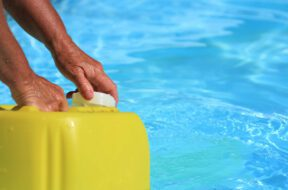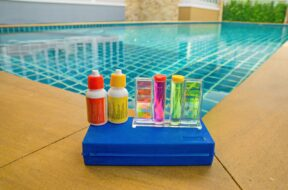# Swimming Pool Volume Calculator

## The Pool Volume Calculator makes it easy to calculate the volume of rectangular, oval, and circular pools quickly.

To keep your swimming pool or hot tub at its best, it’s important that you accurately calculate your water volume. All of the chemical balancing recommendations you receive from the Pool Calculator are based on it. The Pool Volume Calculator is part of the Pool Calculator and is designed to help you estimate your pool volume. The Volume Calculator can calculate volume of rectangular, oval, and round pools quickly. You can calculate the volume of your pool in metric units (liters) or in imperial units (gallons).

## How to Measure your Swimming Pool Volume

To calculate the volume of your pool or spa, you need two measurements: the surface area of the pool, and the average depth.

If your pool is one of the standard shapes available in the pool calculator (rectangle, oval or round), you can measure the length and width of your pool and input into the volume calculator.

To measure the average depth, measure the water in the deep and shallow end of your pool. If the deep and shallow end of your swimming pool are approximately the same surface area, you can estimate the average depth by measuring the water depth in the deep and the shallow add them together, and divide by two. For example, if the shallow end is four feet and the deep end is ten feet, the average depth is (4ft + 10ft)/2 = 7 feet.

## How to properly measure the depth of your swimming pool

When measuring the depth of your pool, it is is important to measure the depth of the water, and NOT the distance from the bottom of the pool to the top of the pool container. Measuring to the top of the pool container can add a significant error, especially if the distance from the top of the water line to the top of the pool or spa is large.

## How to measure the average depth of non standard pools

For most pools, measuring the depth of the water in the deepest part of your pool and the shallowest part of the pool is a pretty good estimate for the average depth. However, if a majority of your swimming pool is either shallow or deep, you need to do a little more work. Measure or make an estimate of the percentage of the pool that is at each depth and adjust the average accordingly.

For example, if the shallow end of your pool is 4 feet deep and is 25% of the pool, and the deep end of the pool is 10 feet deep and is 75% of the pool , you should adjust the average depth of the pool like this:
4 feet*25% + 10 feet*75% = 8.5 feet average depth+

## How to Measure Volume of Complex Pool Shapes

If your pool or hot tub is a complex shape, the best way to calculate the volume is to break the shape into multiple regular shapes and calculate the volume of each as a separate pool. Once you have calculated the volume of these separate pools, add them together to estimate the volume of your entire pool. For example, you can break an “L” Shaped pool into two rectangular pools.

Make sure to separately estimate the average depth of both rectangles if they are different.

## Kidney Shape pools

If you have a kidney-shaped pool, the Pentair Pool Volume calculator can help calculate the volume. For more detail on the math behind the calculation, see the description on the inch calculator website.

## Irregular Pool Shapes

If you have a pool that has an irregular shape that is difficult to measure the surface area, you should do your best to estimate the volume by fitting a shape that doesn’t quite cover the outline of your pool.

For example, if you have an hexagon shaped pool and measure the length across the pool, you can use the circle-shape pool selection in the Pool Volume Calculator and use that measurement for a good approximation of total volume.

Similarly, if the depth of your pool is irregular, start with an estimate that is on the low side. In the example above, it is better to under-estimates the average depth so that the pool volume you use to calculate chemical dosing provides a lower dosing amount.

## General guidelines for calculating Pool Volume

It is better to underestimate your pool volume and add less chemicals to start. It is easier to add more chemicals if the amount you add doesn’t quite get you to the chemical level you are seeking. Fixing an “over treated” swimming pool is a much bigger problem if you add too much chemical. In some cases, the only way to fix it may be to remove water from the pool.

## Conclusion

To summarize, pool volume is the most important factor in taking good care of your pool. The Pool Volume Calculator built into the Pool Calculator can help you with most standard shaped pools. If you have an irregular shaped pool, either break it into multiple smaller pools, or use a shape from the pool volume calculator that best estimates the surface area, making sure to underestimate to begin.

If you have an irregular shaped pool and need help estimating the volume, contact us at [email protected] and we’ll try to help!

### Popular postsCan You Over Shock a Pool?

In this quick guide, we’ll answer the question “can you over shock a pool” and unveil the factors to consider when shocking a pool.Muriatic Acid: Pool Owners Guide

Muriatic acid is one of the most versatile pool chemicals. Read on as we answer the most common muriatic acid pool questions.The Relationship Between pH and Total Alkalinity

Maintaining both pH and total alkalinity in your swimming pool is important for keeping your pool properly sanitized and non-corrosive. Total alkalinity is to pH what cyanuric acid is to free chlorine. Total alkalinity stabilizes pH levels. The ideal pool pH level is 7.4 to 7.6. The ideal total alkalinity level is 80 to 120 ppm.